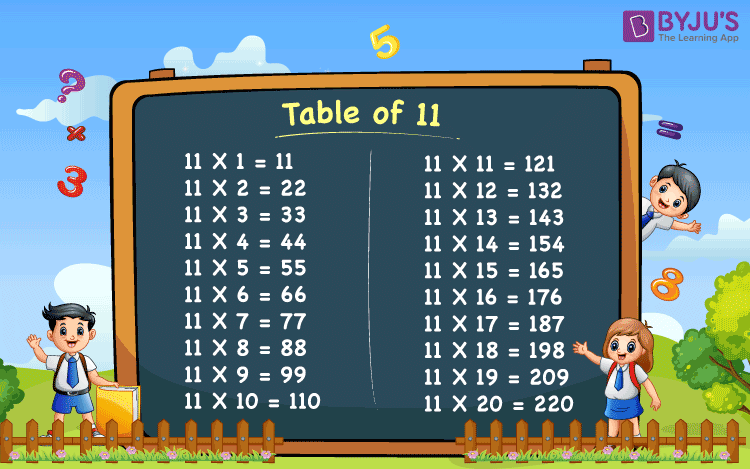# Table of 11

Table of 11 is provided below. The students are suggested to memorize these tables thoroughly for doing quick multiplication of different quantities in day to day calculations and in competitive exams like GMAT and CAT. Also, have a quick revision of tables at BYJU’S, by downloading the table of 11 pdf given below.

## Table of 11 Chart## Table of 11

 11 x 1 = 11 11 x 2 = 22 11 x 3 = 33 11 x 4 = 44 11 x 5 = 55 11 x 6 = 66 11 x 7 = 77 11 x 8 = 88 11 x 9 = 99 11 x 10 = 110 11 x 11 = 121 11 x 12 = 132 11 x 13 = 143 11 x 14 = 154 11 x 15 = 165 11 x 16 = 176 11 x 17 = 187 11 x 18 = 198 11 x 19 = 209 11 x 20 = 220

## Frequently Asked Questions – FAQs

Q1

### What is the 11 times table?

The 11 times table is the multiplication table of 11, which is obtained by multiplying the number 11 with the natural numbers.
Q2

### What is 11 times 5?

11 times 5 is 55. (i.e. 11 × 5 = 55).
Q3

### What is 11 times 3 minus 10?

11 times 3 is 33. (i.e. 11 × 3 = 33).
So, 11 times 3 minus 10 = 33 – 10 = 23 .
Q4

### What is 11 times 20?

11 times 20 is 220. (i.e. 11 × 20 = 220).
Q5

### Calculate 11 times 6 minus 10?

11 times 6 is 66. (i.e. 11 × 6 = 66).
So, 11 times 6 minus 10 = 66 – 10 = 56.
Test your knowledge on Table Of 11